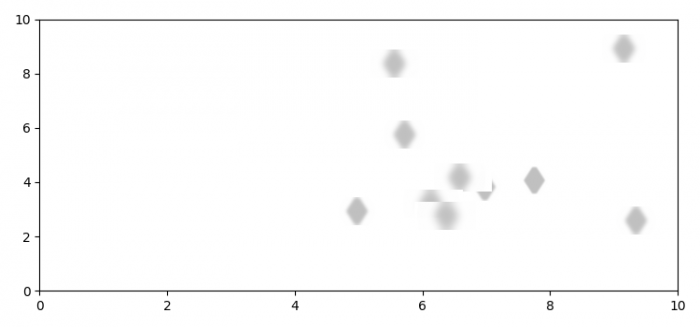# How to plot blurred points in Matplotlib?

To plot blurred points in matplotlib, we can take the following steps −

• Set the figure size and adjust the padding between and around the subplots.

• Create a new figure or activate an existing new figure.

• Add an ax1 to the figure as part of a subplot arrangement.

• First, we can make a marker, i.e., to be blurred.

• Set the X and Y axes scale, turn off the axes.

• Save the marker in a file, and load that image to be plotted after blurred.

• Close the previous figure, fig1.

• Create a new figure or activate an existing figure, fig2.

• Create random data points, x and y.

• Apply Gaussian filter, to make blur, add that artist on the current axes.

• Set the X and Y axes scale, on ax2.

• To display the figure, use show() method.

## Example

import matplotlib.pyplot as plt
from scipy import ndimage
from matplotlib.image import BboxImage
from matplotlib.transforms import Bbox, TransformedBbox
import numpy as np

plt.rcParams["figure.figsize"] = [7.50, 3.50]
plt.rcParams["figure.autolayout"] = True

fig1 = plt.figure()
ax1.plot(0.5, 0.5, 'd', ms=200)
ax1.set_ylim(0, 1)
ax1.set_xlim(0, 1)
plt.axis('off')
fig1.savefig('marker.png')

plt.close(fig1)

fig2 = plt.figure()

x = 8 * np.random.rand(10) + 1
y = 8 * np.random.rand(10) + 1

sigma = np.arange(10, 60, 5)

for xi, yi, sigmai in zip(x, y, sigma):
markerBlur = ndimage.gaussian_filter(marker, sigmai)
bb = Bbox.from_bounds(xi, yi, 1, 1)
bb2 = TransformedBbox(bb, ax2.transData)
bbox_image = BboxImage(bb2,norm=None,origin=None, clip_on=False)
bbox_image.set_data(markerBlur)

ax2.set_xlim(0, 10)
ax2.set_ylim(0, 10)

plt.show()

## OutputUpdated on: 09-Aug-2021

544 Views